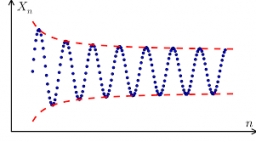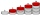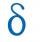# In the 8

In the A. P. 36, 39, 42, …, which term is 276?

Correct result:

n =  81

#### Solution:We would be pleased if you find an error in the word problem, spelling mistakes, or inaccuracies and send it to us. Thank you!## Next similar math problems:

• Insert 4Insert 3 arithmetic means between 3 and 63.
• SequenceBetween numbers 1 and 53 insert n members of the arithmetic sequence that its sum is 702.
• Sequence 3Write the first 5 members of an arithmetic sequence: a4=-35, a11=-105.
• SequenceWrite the first 7 members of an arithmetic sequence: a1=-3, d=6.
• Sequence 2Write the first 5 members of an arithmetic sequence a11=-14, d=-1
• AndrewAndrew had an offer for a job starting at 100000 per year and guaranteeing her to raise of 10000 a year for the next years. Find her salary for the 4th year.
• SeatsSeats in the sport hall are organized so that each subsequent row has five more seats. First has 10 seats. How many seats are: a) in the eighth row b) in the eighteenth row
• AS sequenceIn an arithmetic sequence is given the difference d = -3 and a71 = 455. a) Determine the value of a62 b) Determine the sum of 71 members.
• What is 9What is the 15th term; x1=1.5, d=4.5?
• Negative differenceFind four arithmetic progression members between 7 and -6.
• Insert 3Insert five arithmetic progression members between -7 and 3/2.
• Difference APCalculate the difference of arithmetic progression if the sum of its first 19 members Sn = 8075 and the first member is a1 = 20
• Third memberDetermine the third member of the AP if a4=93, d=7.5.
• What areWhat are the four terms between 4 and 19?
• Find the 19Find the 1st term of the GP ___, -6, 18, -54.
• AP membersWhat is the value of x2, x3, x4, x5…of the terms (of arithmetic progression) when x1 = 8 and x6 = 20?
• Difference AP 4Calculate the difference of the AP if a1 = 0.5, a2 + a3 = -1.1